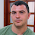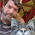## Wednesday, June 29, 2016

### Functional Practice: Euler Problem #1 in F#

Time for a little more coding practice as I'm learning F#. And for that, I'm headed back to Project Euler. Many of these problems lend themselves to functional-style solutions.

I took a look at Euler Problem #1 way back in October 2013 (wow, has it been that long already?) with a solution in Haskell. Since I've already thought about this problem, putting together a solution in F# was pretty simple.

[Update: Collected articles for the first 10 Euler Problems in F# are available here: Jeremy Explores Functional Programming.]

Euler Problem #1
Here is problem 1:
If we list all the natural numbers below 10 that are multiples of 3 or 5, we get 3, 5, 6 and 9. The sum of these multiples is 23. Find the sum of all the multiples of 3 or 5 below 1000.
For the solution in Haskell, we built things up step-by-step. We'll do the same thing here for F#.

Building a Solution
We'll start with the example with the numbers below 10. Once we have a matching output, we'll expand it to work with numbers below 1000.

The first step is to get a list of the natural numbers below 10:

The "[1..9]" creates a list that gives us the natural numbers below 10 -- which translates into integers from 1 to 9 inclusive.

For these examples, I'll just show the input (from an F# script file) and the output (from the F# Interactive window). I prefer using a script file for syntax highlighting and such. But this would work just the same if we typed things into F# Interactive directly.

Piping Forward
The next step is to get the numbers that are multiples of 3 or 5. For this, we'll use the "filter" function:

The "List.filter" function takes 2 parameters. The first parameter is a function used to filter the data. We just have to return a true/false value to determine whether the list item is included in the resulting list. In this case, we use a function that checks to see if "x" is evenly divisible by 3 or 5 (using the modulus operator).

This is very similar to the LINQ "Where" function in C# that takes a "Func<TSource, bool>" (a delegate) as a parameter. The anonymous function we show here is equivalent to a lambda expression in C#.

The last parameter of the "filter" function is the list. In F# (and other functional languages), it is common to have the data parameter as the last parameter. This is a bit different than C# programmers are used to; in C#, we usually put the data parameter as the first parameter (since it's most important).

One reason that we like to have the data parameter last is so that we can easily use pipelining -- where we pass the output of one function as a parameter to another function. In F#, we use the "|>" operator for this. Here's the same function re-arranged to use pipelining:

In this case, we create the list on the first line, then we pipe the output of that list creation to the "List.filter" function. This uses the list as the last parameter of the "filter" function. And this is why we like to have the data parameter as the last parameter of a function -- we can easily pipe the output of a function into the input of another function.

Side note: Another reason to have the "important" parameter last is so that we can do partial application of functions -- but that's not something that we'll be talking about today.

These two ways of calling "List.filter" are equivalent. But when we use pipelining, we give ourselves a very easy way of chaining functions together.

Summing the List
The pipelining doesn't seem too useful when we just have 1 function (well, technically, we have at least 3 functions here, but it looks like 1). But it gets much more interesting when we add more functions. The last step in our problem is to sum the results. We'll just pipe the results to the "List.sum" function:

"List.sum" take a single parameter: a list of values to be added together. When we pipe our filtered list into the "sum" function, we get our expected result of 23.
I really like how easy it is to chain functions together with pipelining. It feels very natural (especially since I'm such a big fan on LINQ in C#).
Completing the Challenge
Now that we've replicated the sample, it's time to see if this works for the full list:

This gives us all the natural numbers below 1000, filters it to values evenly divisible by either 3 or 5, and then sums the result. We've previously verified the result for Euler Problem #1, so we know that this value is correct.

A Reusable Function
As a final step, let's create a function that is reusable. For this, we'll just add a declaration and a parameter:

We called this "euler1" and it takes a parameter "belowThis". This will create a list that ends just below the value passed in. This way, we can call the function with the same values that are in the problem.

Note: We don't have to declare a type for "belowThis" because F# uses type inference pretty much everywhere. Since we're using "belowThis" as an integer (by subtracting one and using it as a value for our list creation), F# determines this is an integer, so we don't have to put that in. Cool stuff (although I sometimes have issues reading code -- I'm hoping I'll get used to it, but I also worry a bit about people who are new to the environment getting frustrated).

For example, this will use all natural numbers below 10:

And here is a call for all numbers below 1000:

We can see that our output values are still correct.

And in case you're wondering, yes, it does fit in a tweet:

Wrap Up
So that takes us through Euler Problem #1 using F#. Nothing too groundbreaking here. And lots of people have solved this problem before. For me, this was a good bit of coding practice, and it helps me get more familiar with F# (which is really my goal here).

The next Euler problem uses the Fibonacci sequence. And creating a "functional" Fibonacci sequence while keeping decent performance has been a problem for me in the past. I might put that on hold a bit while I go through some of my F# books to learn more about the language and constructs. I'm sure there will be a bit of trial and error there.

This type of exploration is how we can learn new things. And even if we're not learning anything new, it helps us get more comfortable with the tools, language, and environment.

Happy Coding!

#### 4 comments:

1.This looks good, the only change I would make to the code above is to use a sequence instead of a list, just to avoid an allocation of memory for a list with belowThis number of elements, while all we need is just to iterate over them. In this case the euler1 becomes something like this:

let euler1 belowThis =
seq{for x in 1 .. (belowThis - 1) do yield x}
|> Seq.filter (fun x -> x % 3 = 0 || x % 5 = 0)
|> Seq.sum

1.And even shorter

seq { 1..(belowThis-1) }

2.Nice, thanks!

3.Very cool! Thanks! Pointing out the advantage of a sequence over a list really makes sense for this scenario. That's very helpful, especially while I'm still learning the basics.